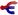# Units

Jump to: navigation, searchThis article is about units of measurement. For the vehicle nicknamed 'The Unit', see Truck.

This page covers various measurement units and derived units used in Satisfactory.

All units should follow SI unit styling guidelines. More details about styling can be found in the style guide.

## Base units

### Time

The base unit of time is a second, or `s`. 60 seconds is one minute, 60 minutes is one hour, obviously.

The duration of one day in Satisfactory is 50 minutes, consisting of a 45-min daytime and 5-min nighttime.

### Item throughput

Item transportation, production and consumption rate is measured in `items per minute` (items/min or ipm), also `parts per minute` (ppm). By default, per minute values are displayed in-game, except for fuel burn time in generators, where the burn duration of one item in seconds is shown instead.

### Distance

The game solely uses meters, `m`, to describe distance, with fractions of it being used in favor of decimeters, centimeters, etc.

### Fluid volume

The volume of fluids is measured in cubic meters, `m3`. Internally, liters are used instead, and to the player, 0.01 m3 is the smallest amount of fluid displayed (= 10 liters).

### Fluid flow rate

The flow rate of fluids is measured in cubic meters per minute, `m3/min`. Some buildings even indicate a direction, with positive values showing inflow and negative values for drainage.

### Head lift

Head lift is measured in unnamed units, where one unit of head lift equals 1 meter. It indicates how high a fluid can be pushed up without its flow rate being reduced below maximum, until stopping entirely.

### Speed

Vehicle speed is measured in kilometers per hour, `km/h` or kph. This can be converted to meter/sec by dividing it by 3.6.

### Power

Power, or rate of energy flow is measured in Megawatts, or `MW`, which is equal to 1,000,000 Watts. This unit is used to measure either building's power production or consumption, as well as equipment or vehicle's fuel burning rate.

Larger amounts of power can be displayed with other prefixes, such as 1,000 MW = 1 GW, and 1 TW = 1,000 GW = 1,000,000 MW. The smallest amount of power both displayed and used in-game is 0.1 MW, which is how much all powered machines consume while their Indicator Light is red or yellow.

### Fuel energy

Every type fuel has a `MJ` value which determines how long it is burned in a generator or a vehicle. For example, one piece of Coal has 300 MJ, and one Coal Generator provides up to 75 MW at 100% clock speed. Generators scale their production based on how much power is being consumed, e.g. when that generator would be connected to a network where 25 MW is being consumed, it would burn three times as slow. One piece of Coal would, therefore, last for 300/25 = 12 seconds at this power usage. If power usage increased all the way to 75 MW (maximum capacity), it would last 300/75 = 4 seconds. Exceeding capacity causes a power trip.

For fluid fuels, the MJ value is for one cubic meter.

## Derived units

### Belt speed

Not to be confused with belt throughput, or item flow rate (see above).

To measure belt speed, build a 48-meters long straight belt (six Foundations) and measure the time taken to transport the player from one end to the other. Divide the distance (48 m) by the time and you got the speed in meters per second, `m/s`. You can convert it into km/h by multiplying it by 3.6.

Higher accuracy measurement can be achieved with longer belts, by chaining multiple belts in a straight line.

### Crafting cycle energy

Similar to fuel energy, the `MJ` required to complete one crafting cycle or to produce one item of that crafting cycle can be calculated using `building power consumption * crafting cycle duration`, e.g. a building consuming 30 MW and completing one production cycle every five seconds consumes 150 MJ per crafting cycle, which equals to half of a piece of Coal being burned in a Coal Generator. If that crafting cycle yields 1 item, it cost 150 MJ, if two items 75 MJ, etc.

Take note as idling buildings constantly consume 0.1 MW while not completing any crafting cycles.

### Power efficiency

Power efficiency is measured in percentage `%`. It is calculated by dividing the net power by gross power.

The gross power is the total amount of power produced by a power generating setup. Net power is gross power minus the internal power consumption used to run the setup, which includes Miners, Water Extractors, Pipeline Pumps, etc.